Case Based Questions (MCQ)

Chapter 11 Class 12 Three Dimensional Geometry
Serial order wise

##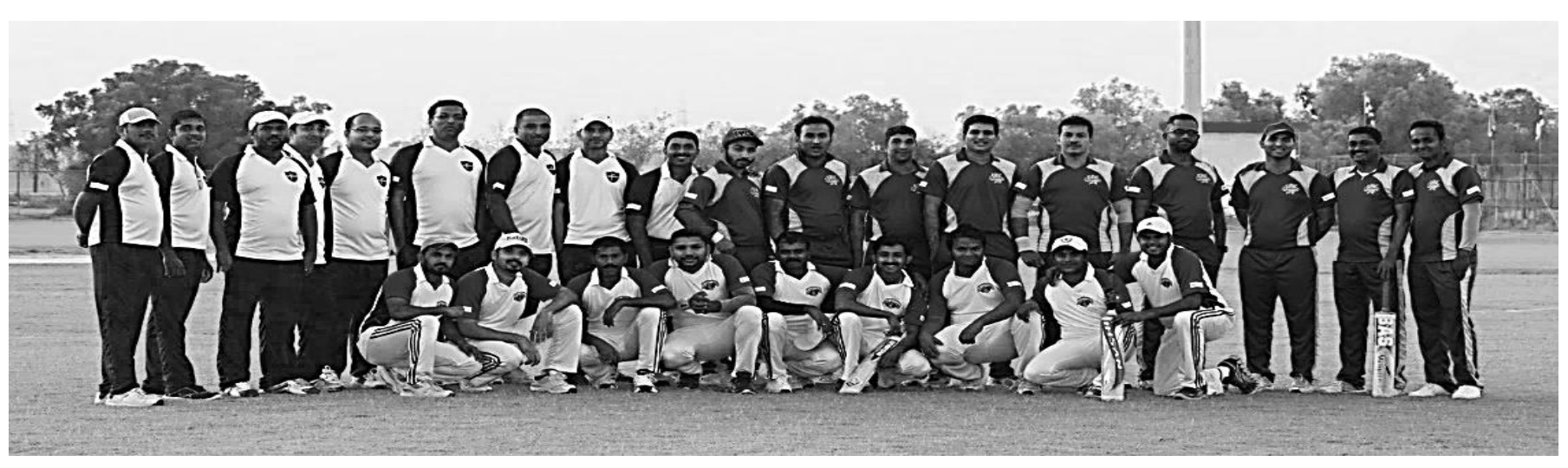googletag.cmd.push(function() { googletag.display('div-gpt-ad-1669298377854-0'); }); Based on the above answer the following: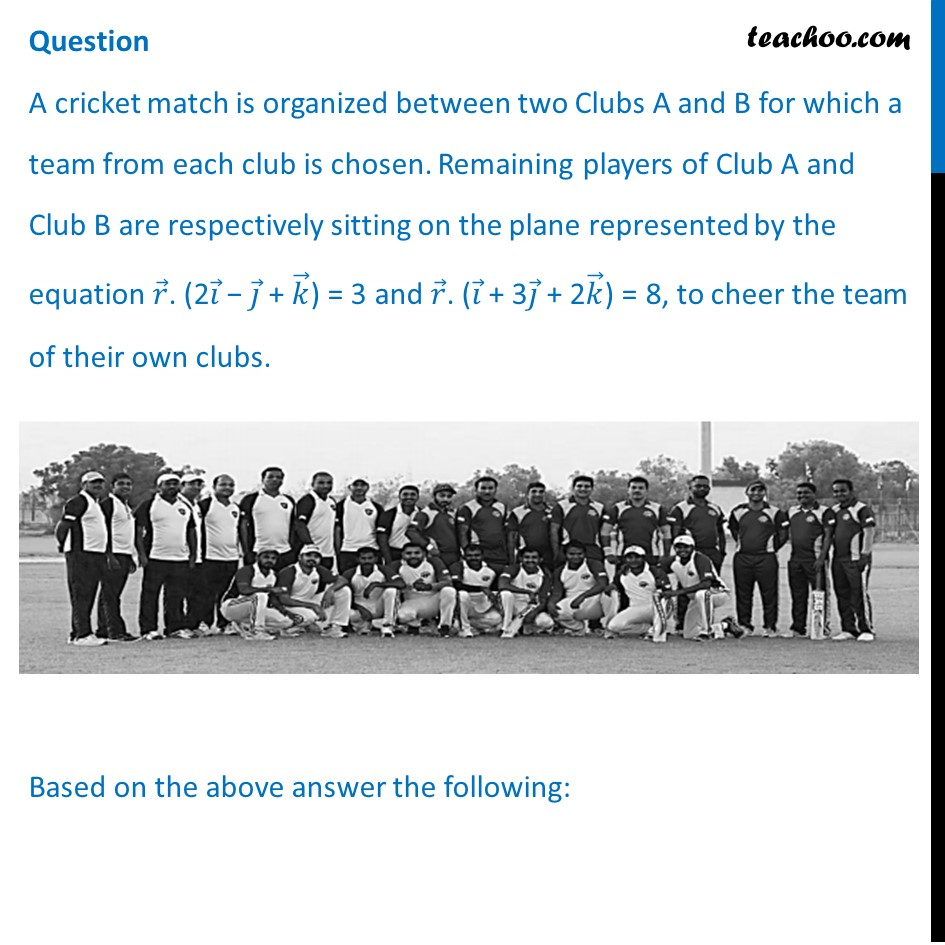## (d) 𝑥 − 𝑦 + 𝑧 = 3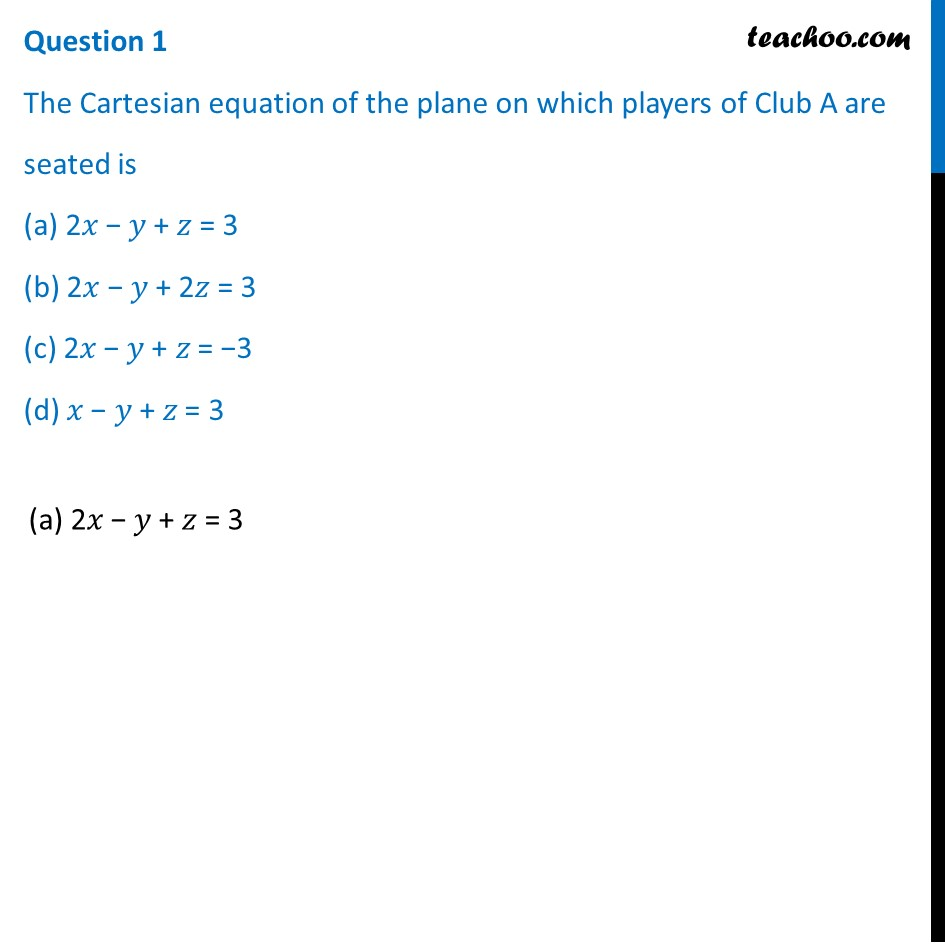## (d) √20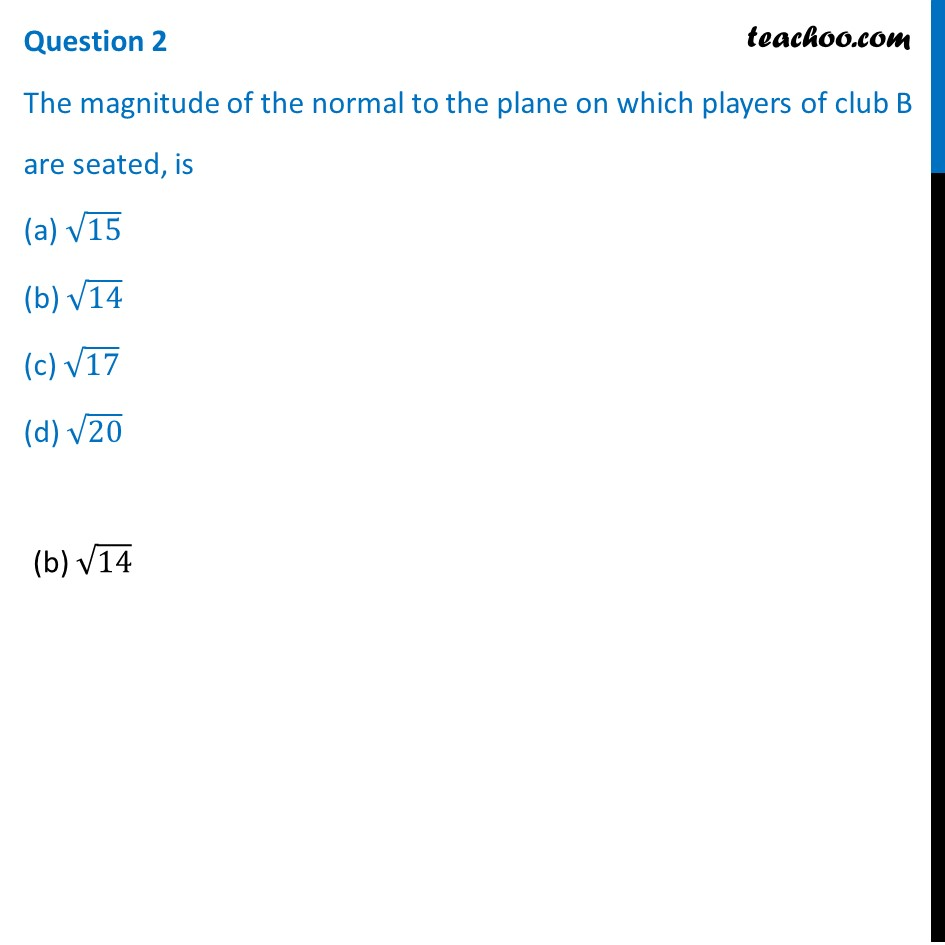## (d) x/8+y/7+z/2=1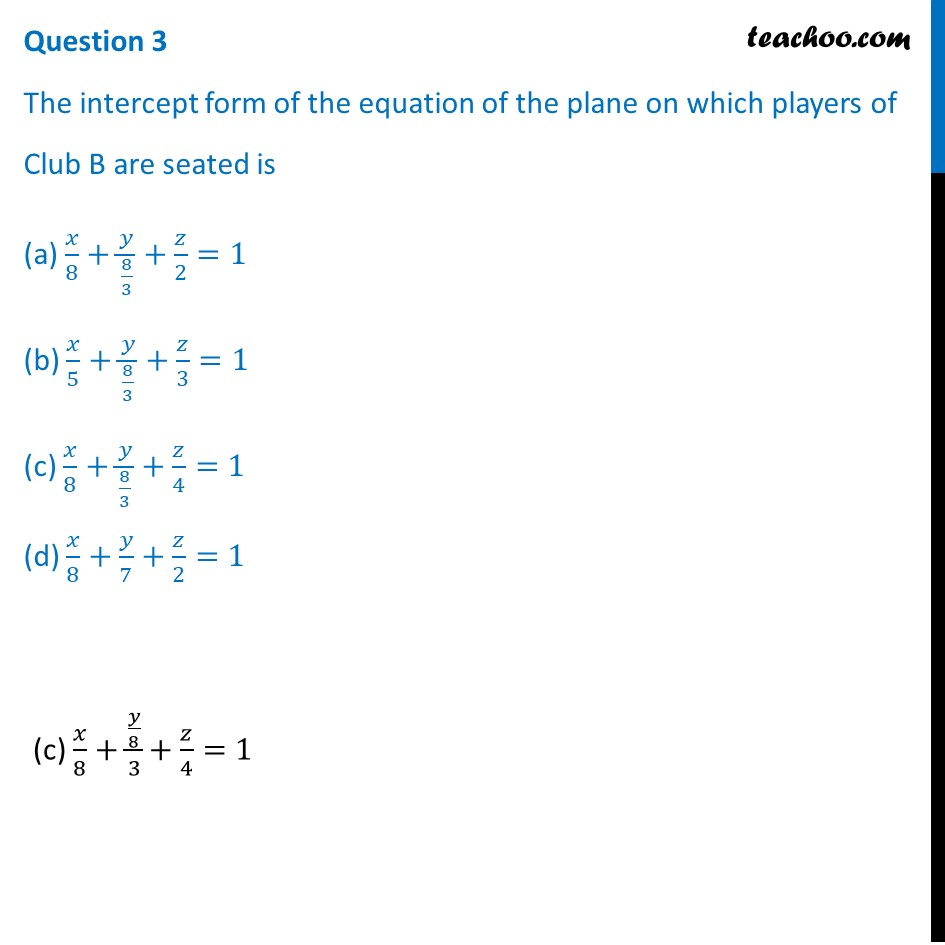## (d) Player sitting at (1, 1, 2)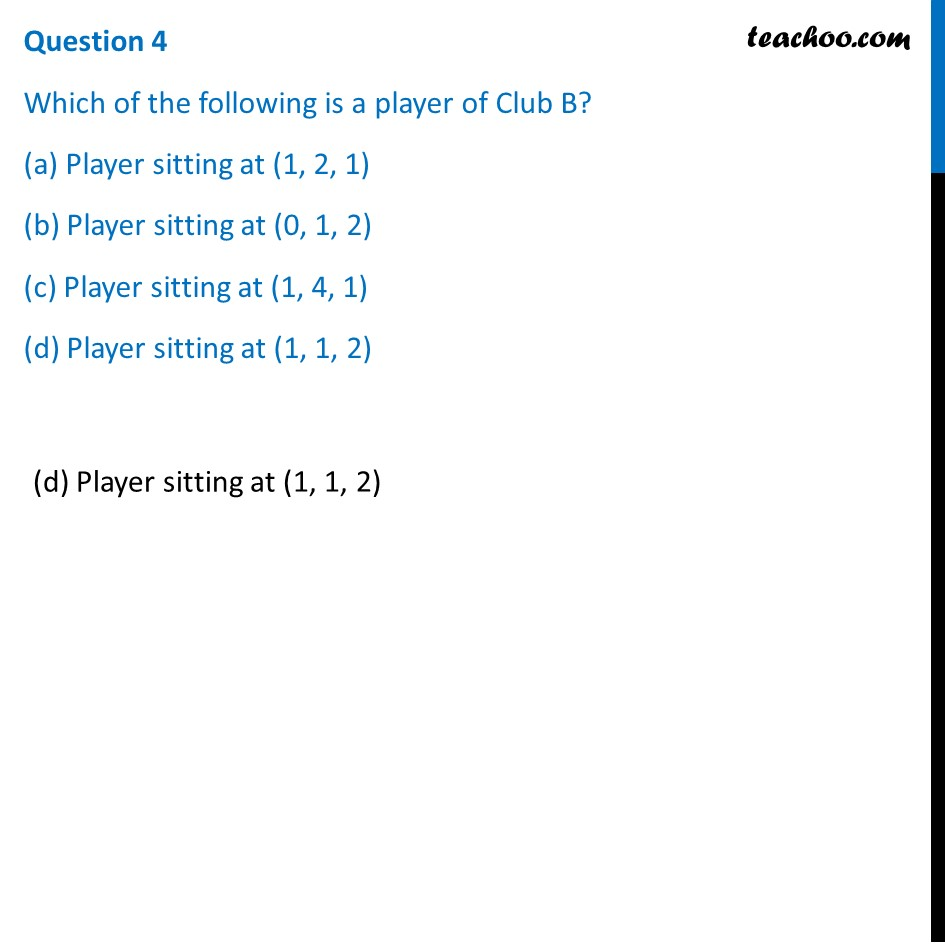## (d) 9/√14 units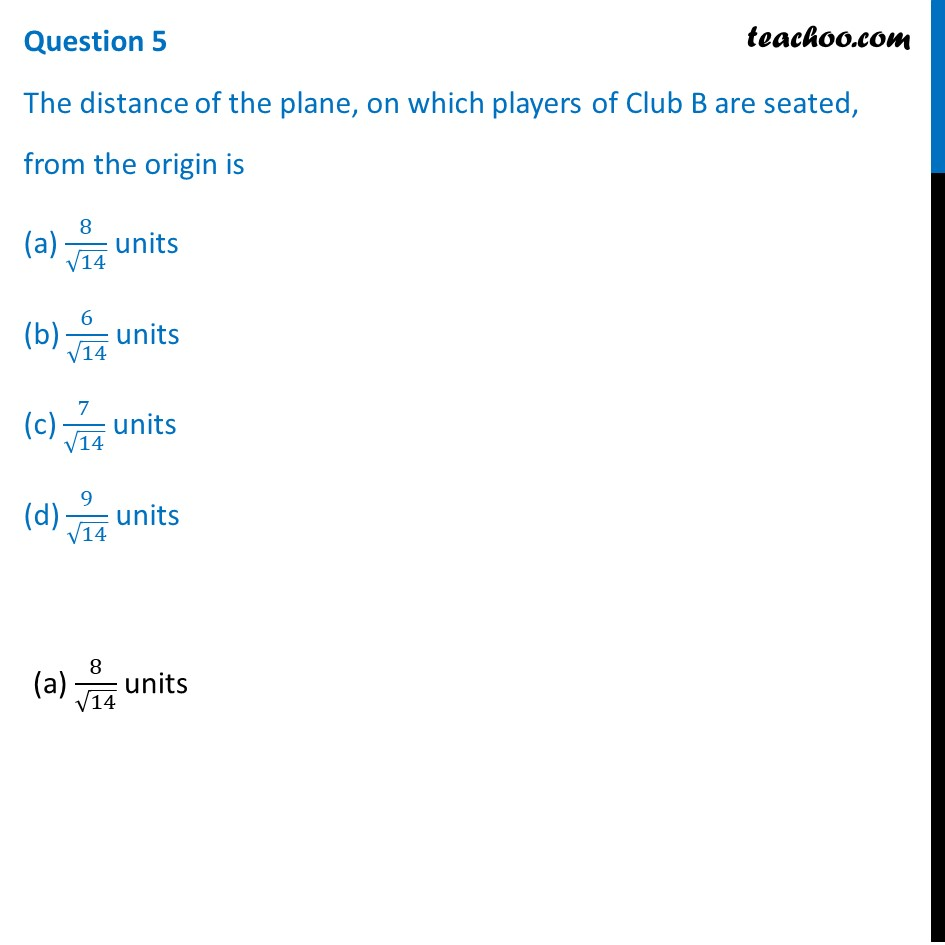Learn in your speed, with individual attention - Teachoo Maths 1-on-1 Class

### Transcript

Question A cricket match is organized between two Clubs A and B for which a team from each club is chosen. Remaining players of Club A and Club B are respectively sitting on the plane represented by the equation 𝑟 ⃗. (2𝑖 ⃗ − 𝑗 ⃗ + 𝑘 ⃗) = 3 and 𝑟 ⃗. (𝑖 ⃗ + 3𝑗 ⃗ + 2𝑘 ⃗) = 8, to cheer the team of their own clubs. Based on the above answer the following:Question 1 The Cartesian equation of the plane on which players of Club A are seated is (a) 2𝑥 − 𝑦 + 𝑧 = 3 (b) 2𝑥 − 𝑦 + 2𝑧 = 3 (c) 2𝑥 − 𝑦 + 𝑧 = −3 (d) 𝑥 − 𝑦 + 𝑧 = 3(a) 2𝑥 − 𝑦 + 𝑧 = 3 Question 2 The magnitude of the normal to the plane on which players of club B are seated, is (a) √15 (b) √14 (c) √17 (d) √20(b) √14 Question 3 The intercept form of the equation of the plane on which players of Club B are seated is (a) 𝑥/8+𝑦/(8/3)+𝑧/2=1 (b) 𝑥/5+𝑦/(8/3)+𝑧/3=1 (c) 𝑥/8+𝑦/(8/3)+𝑧/4=1 (d) 𝑥/8+𝑦/7+𝑧/2=1 (c) 𝑥/8+(𝑦/8)/3+𝑧/4=1 Question 4 Which of the following is a player of Club B? (a) Player sitting at (1, 2, 1) (b) Player sitting at (0, 1, 2) (c) Player sitting at (1, 4, 1) (d) Player sitting at (1, 1, 2)(d) Player sitting at (1, 1, 2) Question 5 The distance of the plane, on which players of Club B are seated, from the origin is (a) 8/√14 units (b) 6/√14 units (c) 7/√14 units (d) 9/√14 units(a) 8/√14 units